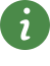# Connectors

Connectors## Library

Modelica/UsersGuide

## Description

The Modelica standard library defines the most important elementary connectors in various domains. If any possible, a user should utilize these connectors in order that components from the Modelica Standard Library and from other libraries can be combined without problems. The following elementary connectors are defined (the meaning of potential, flow, and stream variables is explained in section "Connector Equations" below):

 domain potentialvariables flowvariables streamvariables connector definition icons electricalanalog electrical potential electrical current Modelica.Electrical.Analog.Interfaces Pin, PositivePin, NegativePinelectricalmulti-phase vector of electrical pins Modelica.Electrical.MultiPhase.Interfaces Plug, PositivePlug, NegativePlugelectricalspace phasor 2 electrical potentials 2 electrical currents Modelica.Electrical.Machines.Interfaces SpacePhasorquasistationarysingle phase complex electrical potential complex electrical current Modelica.Electrical.QuasiStationary.SinglePhase.Interfaces Pin, PositivePin, NegativePinquasistationarymulti-phase vector of quasi stationary single phase pins Modelica.Electrical.QuasiStationary.MultiPhase.Interfaces Plug, PositivePlug, NegativePlugelectricaldigital Integer (1..9) Modelica.Electrical.Digital.Interfaces DigitalSignal, DigitalInput, DigitalOutputmagneticflux tubes magnetic potential magnetic flux Modelica.Magnetic.FluxTubes.Interfaces MagneticPort, PositiveMagneticPort,NegativeMagneticPortmagneticfundamentalwave complex magnetic potential complex magnetic flux Modelica.Magnetic.FundamentalWave.Interfaces MagneticPort, PositiveMagneticPort,NegativeMagneticPorttranslational distance cut-force Modelica.Mechanics.Translational.Interfaces Flange_a, Flange_brotational angle cut-torque Modelica.Mechanics.Rotational.Interfaces Flange_a, Flange_b3-dim.mechanics position vector orientation object cut-force vector cut-torque vector Modelica.Mechanics.MultiBody.Interfaces Frame, Frame_a, Frame_b, Frame_resolvesimplefluid flow pressure specific enthalpy mass flow rate enthalpy flow rate Modelica.Thermal.FluidHeatFlow.Interfaces FlowPort, FlowPort_a, FlowPort_bthermofluid flow pressure mass flow rate specific enthalpymass fractions Modelica.Fluid.Interfaces FluidPort, FluidPort_a, FluidPort_bheattransfer temperature heat flow rate Modelica.Thermal.HeatTransfer.Interfaces HeatPort, HeatPort_a, HeatPort_bblocks Real variable Integer variable Boolean variable Modelica.Blocks.Interfaces RealSignal, RealInput, RealOutput IntegerSignal, IntegerInput, IntegerOutput BooleanSignal, BooleanInput, BooleanOutputcomplexblocks Complex variable Modelica.ComplexBlocks.Interfaces ComplexSignal, ComplexInput, ComplexOutputstatemachine Boolean variables (occupied, set, available, reset) Modelica.StateGraph.Interfaces Step_in, Step_out, Transition_in, Transition_outIn all domains, usually 2 connectors are defined. The variable declarations are identical, only the icons are different in order that it is easy to distinguish connectors of the same domain that are attached at the same component.

#### Hierarchical Connectors

Modelica supports also hierarchical connectors, in a similar way as hierarchical models. As a result, it is, e.g., possible, to collect elementary connectors together. For example, an electrical plug consisting of two electrical pins can be defined as:

connector Plug
import Modelica.Electrical.Analog.Interfaces;
Interfaces.PositivePin phase;
Interfaces.NegativePin ground;
end Plug;


With one connect(..) equation, either two plugs can be connected (and therefore implicitly also the phase and ground pins) or a Pin connector can be directly connected to the phase or ground of a Plug connector, such as "connect(resistor.p, plug.phase)".

#### Connector Equations

The connector variables listed above have been basically determined with the following strategy:

1. State the relevant balance equations and boundary conditions of a volume for the particular physical domain.
2. Simplify the balance equations and boundary conditions of (1) by taking the limit of an infinitesimal small volume (e.g., thermal domain: temperatures are identical and heat flow rates sum up to zero).
3. Use the variables needed for the balance equations and boundary conditions of (2) in the connector and select appropriate Modelica prefixes, so that these equations are generated by the Modelica connection semantics.

The Modelica connection semantics is sketched at hand of an example: Three connectors c1, c2, c3 with the definition

connector Demo
Real        p;  // potential variable
flow   Real f;  // flow variable
stream Real s;  // stream variable
end Demo;


are connected together with

   connect(c1,c2);
connect(c1,c3);


then this leads to the following equations:

  // Potential variables are identical
c1.p = c2.p;
c1.p = c3.p;

// The sum of the flow variables is zero
0 = c1.f + c2.f + c3.f;

/* The sum of the product of flow variables and upstream stream variables is zero
(this implicit set of equations is explicitly solved when generating code;
the "<undefined>" parts are defined in such a way that
inStream(..) is continuous).
*/
0 = c1.f*(if c1.f > 0 then s_mix else c1.s) +
c2.f*(if c2.f > 0 then s_mix else c2.s) +
c3.f*(if c3.f > 0 then s_mix else c3.s);

inStream(c1.s) = if c1.f > 0 then s_mix else <undefined>;
inStream(c2.s) = if c2.f > 0 then s_mix else <undefined>;
inStream(c3.s) = if c3.f > 0 then s_mix else <undefined>;